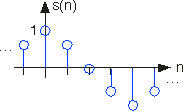# Discrete-Time Signals

So far, we have treated what are known as analog signals and systems. Mathematically, analog signals are functions having continuous quantities as their independent variables, such as space and time. Discrete-time signals are functions defined on the integers; they are sequences. One of the fundamental results of signal theory will detail conditions under which an analog signal can be converted into a discrete-time one and retrieved without error. This result is important because discrete-time signals can be manipulated by systems instantiated as computer programs. Subsequent modules describe how virtually all analog signal processing can be performed with software.

As important as such results are, discrete-time signals are more general, encompassing signals derived from analog ones and signals that aren't. For example, the characters forming a text file form a sequence, which is also a discrete-time signal. We must deal with such symbolic valued signals and systems as well.

As with analog signals, we seek ways of decomposing real-valued discrete-time signals into simpler components. With this approach leading to a better understanding of signal structure, we can exploit that structure to represent information (create ways of representing information with signals) and to extract information (retrieve the information thus represented). For symbolic-valued signals, the approach is different: We develop a common representation of all symbolic-valued signals so that we can embody the information they contain in a unified way. From an information representation perspective, the most important issue becomes, for both real-valued and symbolic-valued signals, efficiency; What is the most parsimonious and compact way to represent information so that it can be extracted later.

## Real- and Complex-valued Signals

A discrete-time signal is represented symbolically as

, where

. We usually draw discrete-time signals as stem plots to emphasize the fact they are functions defined only on the integers. We can delay a discrete-time signal by an integer just as with analog ones. A delayed unit sample has the expression

, and equals one when

.

#### Discrete-Time Cosine SignalFigure 1. The discrete-time cosine signal is plotted as a stem plot. Can you find the formula for this signal?

## Complex Exponentials

The most important signal is, of course, the complex exponential sequence.

## Sinusoids

Discrete-time sinusoids have the obvious form

. As opposed to analog complex exponentials and sinusoids that can have their frequencies be any real value, frequencies of their discrete-time counterparts yield unique waveforms only when

lies in the interval

. This property can be easily understood by noting that adding an integer to the frequency of the discrete-time complex exponential has no effect on the signal's value.

This derivation follows because the complex exponential evaluated at an integer multiple of

equals one.

## Unit Sample

The second-most important discrete-time signal is the unit sample, which is defined to be

#### Unit Sample

Examination of a discrete-time signal's plot, like that of the cosine signal shown in Figure, reveals that all signals consist of a sequence of delayed and scaled unit samples. Because the value of a sequence at each integer

is denoted by

and the unit sample delayed to occur at

is written

, we can decompose any signal as a sum of unit samples delayed to the appropriate location and scaled by the signal value.

This kind of decomposition is unique to discrete-time signals, and will prove useful subsequently.

Discrete-time systems can act on discrete-time signals in ways similar to those found in analog signals and systems. Because of the role of software in discrete-time systems, many more different systems can be envisioned and “constructed” with programs than can be with analog signals. In fact, a special class of analog signals can be converted into discrete-time signals, processed with software, and converted back into an analog signal, all without the incursion of error. For such signals, systems can be easily produced in software, with equivalent analog realizations difficult, if not impossible, to design.

## Symbolic-valued Signals

Another interesting aspect of discrete-time signals is that their values do not need to be real numbers. We do have real-valued discrete-time signals like the sinusoid, but we also have signals that denote the sequence of characters typed on the keyboard. Such characters certainly aren't real numbers, and as a collection of possible signal values, they have little mathematical structure other than that they are members of a set. More formally, each element of the symbolic-valued signal

takes on one of the values

which comprise the alphabet

. This technical terminology does not mean we restrict symbols to being members of the English or Greek alphabet. They could represent keyboard characters, bytes (8-bit quantities), integers that convey daily temperature. Whether controlled by software or not, discrete-time systems are ultimately constructed from digital circuits, which consist entirely of analog circuit elements. Furthermore, the transmission and reception of discrete-time signals, like e-mail, is accomplished with analog signals and systems. Understanding how discrete-time and analog signals and systems intertwine is perhaps the main goal of this course.Swipe left and right to change pages.